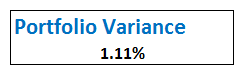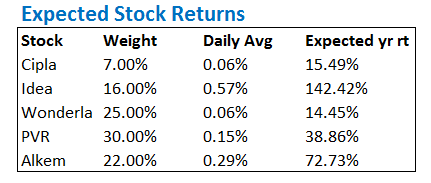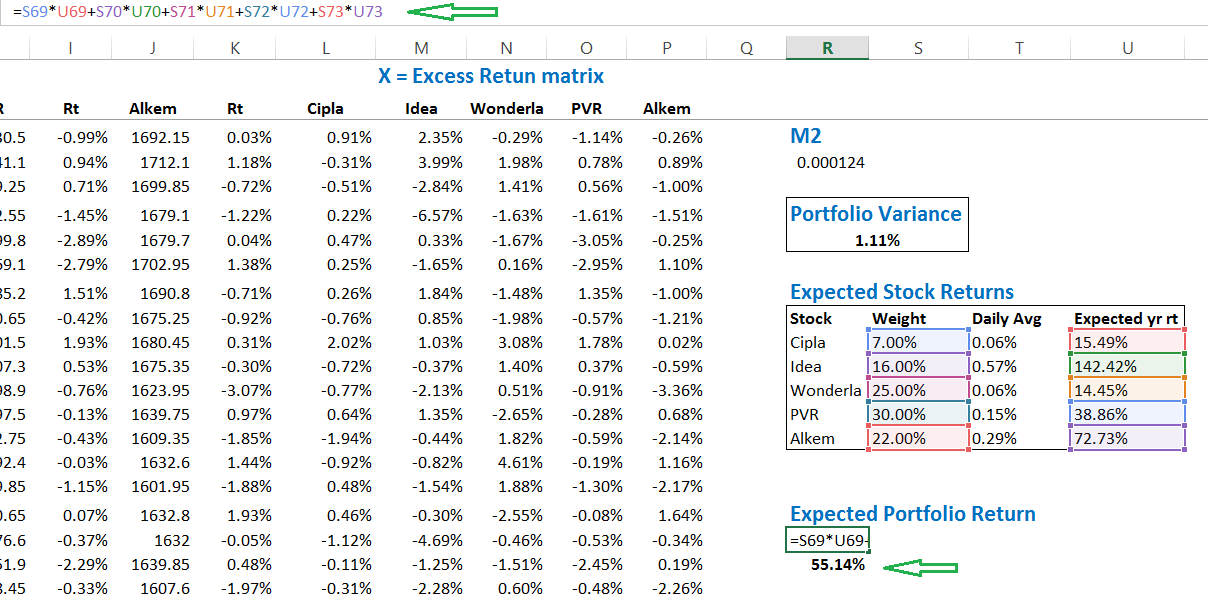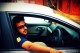Module 9 Risk Management & Trading Psychology

Chapter 7

# Expected Returns

## 7.1 – Expected returnsThe next two chapter will be very insightful, especially for people who have never been familiar with portfolio techniques. We will venture into the realms of expected return framework and portfolio optimization. Portfolio optimization in particular (which we will discuss in the next chapter) is like a magic wand, it helps you decide how much to invest in a particular stock (within a portfolio) so that you achieve the best possible results in terms of risk and return. These are topics which the high priests of finance prefer to keep for themselves, but today we will discuss them here and truly work towards democratizing quality financial knowledge.

But please note, to best understand the discussion here, you need to have a sense of all the things we have discussed over the previous couple of chapters. If you have not read them yet, please, I’d urge you to read them first. This is good quality information and you would be a better market participant if you simply spent few hours reading them. The excel sheet used here is a continuation of the one used in the previous chapters.

So assuming you are all set, let us get started.

It is time we put the portfolio variance to good use. To begin with let us take a good look at the portfolio variance number calculated in the previous chapters –What does this number tell you?

The number gives you a sense of the degree of the risk associated with the portfolio. Remember, we worked on the daily data, hence the Portfolio Variance of 1.11% represents risk on a daily basis.

Risk or variance or volatility is like a coin with two faces. Any price movement below our entry price is called risk while at the same time, the same price movement above our entry price is called return. We will soon use the variance data to establish the expected range within which the portfolio is likely to move over the year. If you’ve read the Options module you will probably know where we are headed.

However, before doing that, we need to figure out the expected return of the portfolio. The expected return of the portfolio is simply, the grand sum of the average return of each stock, multiplied by its weight and further multiplied by 252 (number of trading days). In simple terms, we are scaling the daily returns to its annual return, and then scaling it according to the investment we have made.

Let us calculate the expected return for the portfolio that we have, I’m sure you will understand this better. To begin with, I’ve lined up the data as follows –The first 3 columns are fairly easy to understand I suppose. The last column is simply the multiplication of the daily average return by 252 – this is a step to annualize the return of the stock.

For example (Cipla) – 0.06% * 252 = 15.49%.

What does this mean? For a moment assume, I have invested all the money in just Cipla and not other stocks, then the weight of Cipla would be 100% and I can expect a return fo 15.49%. However, since I’ve invested only 7% of my capital in Cipla, the expected return from Cipla would be –

Weight * Expected Return

= 7% * 15.49%

=1.08%

We can generalize this at the portfolio level to get the expected return of the portfolio –Where,

Wt = Weight of each stock

Rt = Expected annual return of the stock

I’ve applied the same formula for the 5 stock portfolio that we’ve got, and here is what we have –At this stage, we have arrived at two extremely important portfolio parameters. They are the expected portfolio return which is 55.14% and the portfolio variance which is 1.11%.

In fact, we can scale the portfolio variance to represent the annual variance, to do this we simply have to multiply the daily variance by Square root of 252.

Annual variance =

= 1.11% * Sqrt (252)

= 17.64%.

We will keep both these important numbers aside.

It is now time to recall our discussion on normal distribution from the options module.

I’d suggest you quickly read through the ‘Dalton board experiment’ and understand normal distribution and how one can use this to develop an opinion on future outcome. Understanding normal distribution and its characteristics is quite crucial at this point. I’d encourage you to read through it before proceeding.

Portfolio returns are normally distributed, I’ll skip plotting the distribution here, but maybe you can do this as an exercise. Anyway, if you do plot the distribution of a portfolio, you are likely to get a normally distributed portfolio. If the portfolio is normally distributed, then we can estimate the likely return of this portfolio over the next 1 year with certain degree of confidence.

To estimate the return with certain degree of confidence we simply have to add and subtract the portfolio variance from the expected annualized return. By doing so we will know how much the portfolio will generate or lose for the given year.

In other words, based on normal distribution, we can predict (although I hate using the word predict in markets) the range within which the portfolio is likely to fluctuate. The accuracy of this predication varies across three levels.

• Level 1 – one standard deviation away, 68% confidence
• Level 2 – Two standard deviation away, 95% confidence
• Level 3 – Three standard deviation away,99% confidence

Remember, variance is measured in terms of standard deviation. So it is important to note that the annualized portfolio variance of 17.64% is also the 1 standard deviation.

So, 17.64% represents 1 standard deviation. Therefore, two standard deviation is 17.64% * 2 = 35.28% and 3 standard deviation would be 17.64% * 3 = 52.92%.

If you are reading this for the first time, then yes, I’d agree it would not be making any sense. Hence it is important to understand normal distribution and its characteristics. I’ve explained the same in the options chapter (link provided earlier).

## 7.2 – Estimating the portfolio range

Given the annualized variance (17.64%) and expected annual return (55.14%), we can now go ahead and estimate the likely range within which the portfolio returns are likely to vary over the next year. Remember when we are talking about a range, we are taking about a lower and upper bound number.

To calculate the upper bound number, we simply had to add the annualized portfolio variance to the expected annual return i.e 17.64% + 55.15% = 72.79%. To calculate the lower bound range we simply have to deduct the annualized portfolio variance from the expected annual return i.e 55.15% – 17.64% = 37.51%.

So, if you were to ask me – how are the returns likely to be if I decide to hold the 5 stock portfolio over the next year, then my answer would be that the returns are likely to fluctuate between +37.51% and +72.79%.

Three quick question may crop up at this stage –

1. The range suggests that the portfolio does not lose money at all, how is this even possible? In fact, the worst case scenario is still a whopping +37.51%, which in reality is fantastic.
1. True, I agree it sounds weird. But the fact is, the range calculation is statistics based. Remember we are in a bull market (April – May 2017, as I write this), and the stocks that we have selected have trended well. So quite obviously, the numbers we have got here is positively biased. To get a true sense of the range, we should have taken at least last 1 year or more data points. However, this is beside the point here – remember our end objective is to learn the craft and not debate over stock selection.
2. Alright, I may have convinced you on the range calculation, but what is the guarantee that the portfolio returns would vary between 37.15% and 72.79%?
1. As I mentioned earlier, since we are dealing with level 1 (1 standard deviation), the confidence is just about 68%.
3. What if I want a higher degree of confidence?
1. Well, in this case you will have to shift gears to higher standard deviations.

Let us do that now.

To calculate the range with 95% confidence, we have to shift gears and move to the 2nd standard deviation. Which means we have to multiply the 1 standard deviation number by 2. We have done this math before, so we know the 2nd SD is 35.28%.

Given this, the range of the portfolio’s return over the next 1 year, with 95% confidence would be –

Lower bound = 55.15% – 35.28% = 19.87%

Upper bound = 55.15% + 35.28% = 90.43%

We can further increase the confidence level to 99% and check the return’s range for 3 standard deviation, recall at 3 SD, the variance is 52.92% –

Lower bound = 55.15% – 52.92%% = 2.23%

Upper bound = 55.15% + 52.92% = 108.07%

As you may notice, the higher the confidence level, the larger the range. I’ll end this chapter here with a set of tasks for you –

1. Plot the frequency distribution for this 5 stock portfolio – observe the distribution, check if you see a bell curve
2. We are dealing with the range for a year, what if you were to estimate the range for 3 months, or maybe 3 weeks? How would you do it?

It will be great if you can attempt these tasks, please do leave your thoughts in the comment box below.

### Key takeaways from this chapter

1. The returns of the portfolio is dependent on the weights of the individual stocks in the portfolio
2. The calculate the effect of an individual stock on the overall portfolio’s return, one has to multiply the average return of the stock by its weight
3. The overall expected return of the portfolio is grand sum of the individual stock’s returns (which is scaled by its weight)
4. The daily variance can be converted to annualized variance by multiplying it by square root of 252
5. The variance of the portfolio which we calculate is by default the 1st standard deviation value
6. To get the 2nd and 3rd SD, we simply have to multiply it by 2 and 3
7. The expected return of the portfolio can be calculated as a range
8. To get the range, we simply have to add and subtract the variance from the portfolio’s expected return
9. Each standard deviation comes with a certain confidence level. For higher confidence level, one has to look at moving higher standard deviation
Module 9

#### Chapters

1.Ar Arbit says:

Thanks a lot for initiating such a nice Varsity. I would like to know, do you have any plan to launch a mobile app for Varsity? That would be a great interface to learn on the go.

•Karthik Rangappa says:

Varsity is already mobile friendly, the site itself adjusts to the mobile device.

2.Yogendra says:

Great

•Karthik Rangappa says:

Cheers!

3.Sumil says:

Thanks a lot for such a great initiative. I’ve never seen so much information about finance in such easy to unterstand language at one place.
This might be a slightly uncorrelated quoestion but I want to start learning algo trading and quant finance.

I’ve engineering background and have 6-7 years experience in investing in stock markets. Is it feasible to someone like me to get started in these fields, or is it a domain of those have advanced degrees in math, comp sc. etc(as many would have you believe).
What is the best place to get started. Want to set up my own algo trading desk someday.

•Karthik Rangappa says:

Quants is a highly specialized field and is heavily dependent on maths. I’d suggest you check out CQF certification to get an idea of how intense this can get.

•SUMIL says:

Went to CQF course. The course is quite on expensive side.
Is there any other cheaper course that teaches this from Indian markets perspective.
Do we need to know advanced math if we just want to do algo trading from home?

•Karthik Rangappa says:

Math is good to know…and if Qants is the direction you want to head, then there are no other good options.

4.pranay dhande says:

•Karthik Rangappa says:

Sure, will do as soon as the current module is through.

5.S KUMAR says:

why not you provide ADX indicator in ZERODHA Pi.

•Karthik Rangappa says:

Its there.

6.Hari prabhu says:

Then thank you for making such a clear theory on all the topics..
Now I feel I have wasted my 5 years of trading session with other brokerages..
Really appreciate your team for all your work.. User friendly system !!! Simply great..
I have one question, after knowing all chard reading method I am confused applying all of them on intraday trading.. Could you please suggest few charts which I can follow up for intraday ?

7.Gautam says:

Hi,Kartik I am learning a lot from you.Every thing you provide here in an unique and easy way..i would like to ask you a question here..you have used portfolio variance instead standard deviation because standard deviation would give us a wide range,am I right?
Can I know,where should I exactly use standard deviation instead variance and variance instead std..?because in option the same approach was done with standard deviation to use confidence level.Do you mean for single stock std used and for over all portfolio variance used to interpret confidence level.

•Karthik Rangappa says:

For a single stock, its best to use the standard deviation…but like you mentioned, for portfolios use the port variance.

8.Himanshu Singhal says:

I think the values calculated are wrong here.
I have a screenshot of the error but I will try to explain it here.
The very first thing is that the average taken of each individual stocks is wrong.

Example: if we look at the excel as shared in this varsity (downloadable attachment) shows average of stocks as thus
Cipla in Cell C 131 = 0.06%, Idea in cell E131 = 0.57%, Wonderla in cell G131 = 0.06%, PVR in cell I131 = 0.15% and Alkem in cell K131 = 0.29%.

However, actual values upon calculation are Cell C 131 = 0.02%, cell E131 = 0.26%, cell G131 = -0.05%, cell I131 = 0.12% and cell K131 = 0.23%.
The Portfolio Variance is 1.11% and Annualized = 17.57%
Expected return = 29.70%

Confidence Interval as follows
SD 1 SD 2 SD 3 SD
Upper 47.27% 64.84% 82.41%
Lower 12.13% -5.45% -23.02%

*The error was in calculating the average at the first step i.e for example for Cipla = average(C51:C131) instead should be average(C5:C131).
The same for all the other 4 stocks.

Hope you will ratify the error and repost.

•Karthik Rangappa says:

Absolutely agree, Himanshu. I’ve missed calculating the average for all cells but by mistake calculated only for few cells. Someone pointed this earlier as well (check the comments). I; ve not really rectified this as these calculations would have to be changed across many chapters. I regret this mistake.

However, I’d suggest you download the excel and make that small correction, the model will self-correct.

9.Raghuram says:

Hi Karthik,
It is said that more than 90% traders lose money in intraday. In my opinion the reason could be due to low risk and high reward ratio strategy. What, if the ratio is reversed. For example, I picked up a stock after studying it carefully and I buy Qty- 1 for 100/- and put stop loss at 94/- and target at 102/-. This way, if price goes down 2 or 3 points my stop loss would not be triggered and and target would be achieved when trend changes. But, If I put stop loss at 98/- and target at 105/- then chances of stop loss triggering are extremely high than hitting the target. Needless to say one must be mentally ready for 6/- loss for a risk of 2/-. Do you agree with my point ?

•Karthik Rangappa says:

I’d attribute lack of discipline as the main cause of the losses. Risk reward etc can be adjusted based on position sizing.

10.Subham Agarwal says:

Hi Sir,

In the above discussion, our expected return from Cipla is 15.49% (leave errors). But the base price consiered to calculate this return changes on daily basis.

Suppose, if on 1.10.17, Cipla’s closing price is 500 and on 2.10.17, it is 400, then our return is (20%). Suppose on 3.10.17, closing price is again 500, then our return would be 25%. If we consider the above formula, our avg daily return would come 2.5%, but in actuals, our return is 0% and vice-versa.

Therefore, our return of 15.49% would be baseless, when calculated on our purchase price.

•Karthik Rangappa says:

Well, for this exact reason you may want to consider taking log returns!

11.Harsh says:

Sir, thank you for your great work.
My question is that, standard deviation and variance are different things.So why you have used variance instead of standard deviation.
The 68-95-99 rule applies to standard deviation,I think…

•Karthik Rangappa says:

They both are the same – square root of variance yields standard deviation.

•Harsh says:

Ok..but have we done the square root in the above example..,if not, why??

•Karthik Rangappa says:

I guess we have.

•Harsh says:

And sorry to bother you..but I have one more question,I have read somewhere that stock prices do not follow normal distribution. Please clear my confusion on this.
Is it like that ,that stock prices do not follow normal distribution but their daily return follow, normal distribution??

•Karthik Rangappa says:

Yes, stock prices don’t – remember the prices can trend either upwards or downwards for an extended period of time. But the stock returns does, somewhat, display a normal distribution.

12.Neha says:

Very Helpful. Pls document this in PDF format.

•Karthik Rangappa says:

13.Anil N says:

Hi Karthik,
Regarding Return of the stock for past data will available in NSE, If i need the Dividend information of past data, where can i get with free. Whether it will available for free?

•Karthik Rangappa says:

You will have to dig up the dividend history. This is available on money control I guess.

14.Sri says:

Hello sir
In the 44th page of 7th chapter, the daily average return is different from the daily average return that we got in chapter 3 14th page. I have downloaded the excel sheet that has been used in 7th chapter. It shows that you have taken average from C51 to C130 while you took C1 to C130 in chapter 3. Is there a reason behind this or this is a calculation mistake?

•Karthik Rangappa says:

I must have taken the latest data set. The technique remains the same though.

15.Shubham D says:

Hi Karthik,

Just wanted to confirm the answer for your question – How would we estimate the same for range of 3 months/ 3 weeks?
We would convert daily returns to appropriate range and proceed with similar calculations.

Is that correct?

Thanks

•Karthik Rangappa says:

Yes, you can convert the daily return to the desired time frame.

16.Rajat says:

Sir I have calculated annual return of Infosys and I got 40% negative return but when I use kite platform the info of Infosys shows positive return of 18%. Have I calculated it wrong?.Because it cannot give positive return as price of share has dropped over the period.

•Karthik Rangappa says:

Maybe you should double check the dates again.

•Rajat says:

I downloaded data from NSE of 365 days and I doubled check regarding dates.On 23 May 18 price of share is 1184.9 and on 22May 19 it is 709.75.So 709.75/1184.9-1 = – 40.10% and info says 20.18% 1 year return on kite platform.

•Karthik Rangappa says:

Ah, then you need to clean up the data for bonus/split 🙂

•Rajat says:

Thank u so much sir.So for calculating portfolio variance also I have to clean up bonus split

•Karthik Rangappa says:

Yes, thats right.

17.PRAKUL says:

my annual ROI is 107% average maintained. Now, my next target is 150%. 🙂

•Karthik Rangappa says:

Good luck!

18.koushik says:

sir ,
can we take it as ,till 5th chapter (risk part 4) as one method and 6th and 7th chapters as another method to find out portfolio variance and expected returns?
were in 6th and 7th chapters we can find out portfolio variance and expected returns from daily returns of the weights of the stocks?

•Karthik Rangappa says:

Hmm, maybe you can 🙂

19.koushik says:

thank you sir

•Karthik Rangappa says:

Welcome!

20.srikanth says:

wow

21.Guru Prasad says:

Great module Mr. Karthik. Firstly id like to commend you on the clarity of content that you ve provided.
I have a question: to plot the freq distributioon curve, what variables must i consider/ is it the returna and date?

•Karthik Rangappa says:

Thanks, Guru.

You need to consider returns and the frequency of returns i.e. the number of times it occurs.

22.Ronak Parekh says:

Good Day,

Thank you so much for this amazing content!

Just wanted to know, being a long term investor (3-5 years) approx, is it okay to gauge the risk by considering weekly avg. returns instead of daily returns?? or will it cause too much change in the confidence levels ??

23.SANKET says:

Sir,to calculate expected returns you multiplied daily avg with 252 but instead it should be with SQRT(252)!!As discussed in earlier chapters!!! Would you enlighten me on this??
You are the best source of knowledge for stock markets. Really Appreciable.

•Karthik Rangappa says:

No, return should not be multiplied by Sqrt of time, that’s only for volatility.

24.vinayak says:

So here we forecast the future based on historical data
Is this right or wrong?

•Karthik Rangappa says:

Yes, thats right.

25.Ganesh says:

Hi,

My question is, say I do this exercise and now I can statistically say with 95% accuracy that my portfolio returns would be between 19 and 90% but the range is so wide here. How does this number, I mean this range, help me in making a real life decision about my portfolio in any way? Thanks in advance :’)

•Karthik Rangappa says:

Ganesh, clearly the range is wide and quite useless. These are times you can reduce the SD to say 66% and see if you can deal with the numbers.

15 chapters

22 chapters

16 chapters

13 chapters

24 chapters

14 chapters

7 chapters

19 chapters

16 chapters